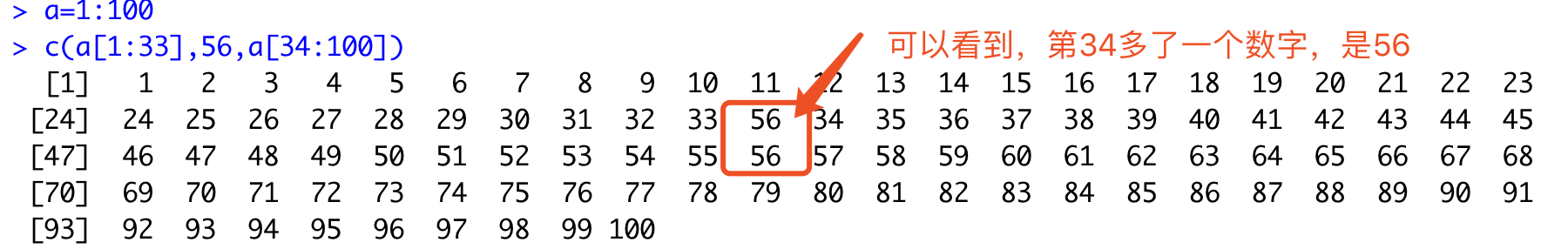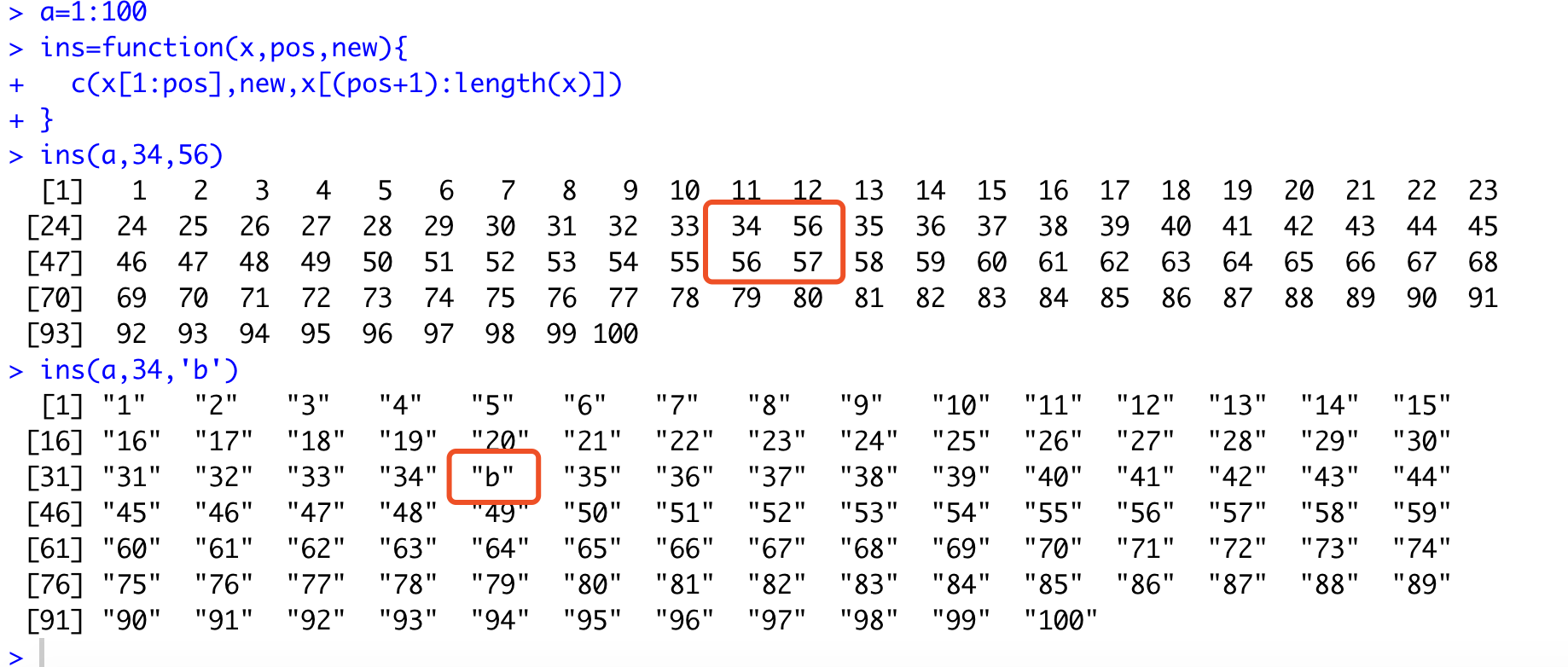# 使用R语言在向量的任何位置插入任何元素

``````a=1:100
c(a[1:33],56,a[34:100])
````````````a=1:100
ins=function(x,pos,new){
c(x[1:pos],new,x[(pos+1):length(x)])
}
ins(a,34,56)
ins(a,34,'b')
``````